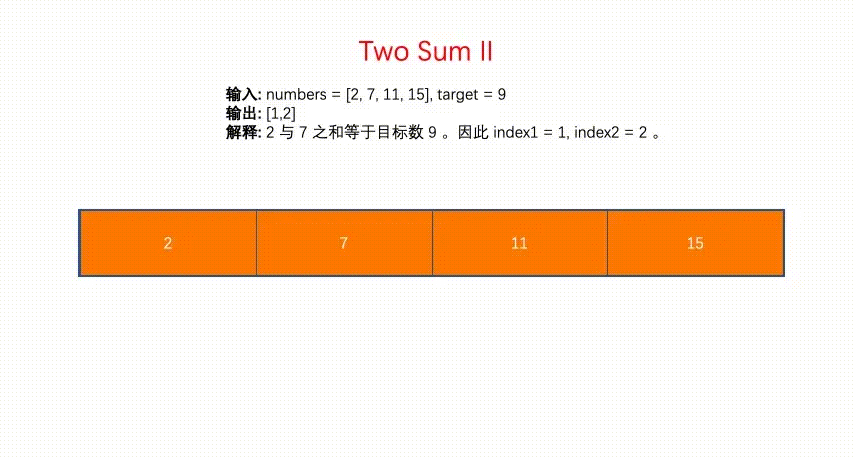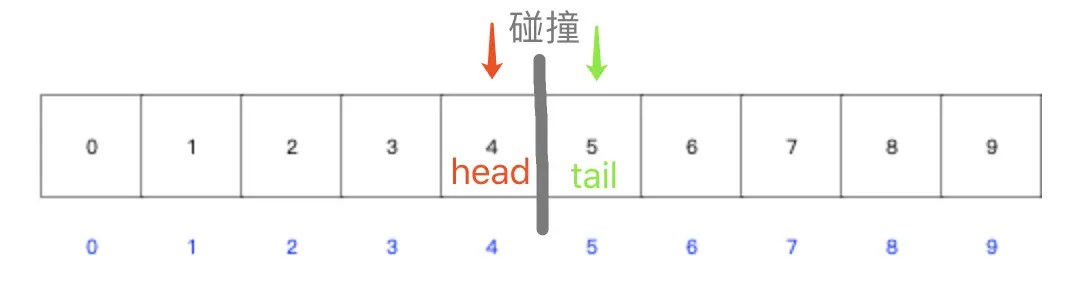# Discover the beauty of the algorithm-the collision of double pointers

Posted Jun 5, 20206 min read• What is a collision pointer?

• First acquaintance
• Algorithm diagram
• Collision process diagram
• Array and collision pointer in JavaScript

• How to define collision pointer in js?
• Implement a minimalist collision pointer
• Leetcode collision pointer solution problem

1. Integer inversion(easy)
1. Palindrome(easy)
1. Remove elements(easy)
1. Verify the palindrome string(easy)
1. Two-number II-input ordered array(easy)
1. Reverse binary bits(easy)
1. Reverse string(easy)
1. Reverse vowels in strings(easy)
1. The container with the most water(medium)

### What is a collision pointer?

#### First acquaintance

• Collision pointer is one of the double pointer algorithm.
• The collision pointer iterates the array from both ends to the middle. One pointer starts at the beginning and the other starts at the end.
• The termination condition of the colliding pointer is that the two pointers meet.
• Collision pointers are often used to sort arrays.

#### Algorithm diagram#### Collision process diagram

1. Two-number II-input ordered array(easy) collision process diagram.
Blue pointer:head pointer Red pointer:tail pointer Termination condition:collision between head and tail pointer### Array and collision pointer in JavaScript

#### How to define collision pointer in js?

##### Naming

The head and tail pointers can be named:

• `i, j`
• `head, tail`
• `start, end`
##### Initial value

• Tail pointer:Array length minus one

let arr = [1, 7, 5, 2];
let tail = arr.length -1;

#### Implement a simple collision pointer``````var arr = new Array(10).fill(0).map((num,i)=>num+i);
var i =0;
var j = arr.length-1;
while(i<j){
i++;
j--;
}
var collision = [i-1, j + 1]
var tip = `Array's head and tail had a collision between \${collision} and \${collision}`;
console.log(tip); //Array's head and tail had a collision between 4 and 5``````

### leetcode collision pointer solution problem

1. Integer inversion(easy)
1. Palindrome(easy)
1. Remove elements(easy)
1. Verify the palindrome string(easy)
1. Two-number II-input ordered array(easy)
1. Reverse binary bits(easy)
1. Reverse string(easy)
1. Reverse vowels in strings(easy)
1. The container with the most water(medium)

#### 7. Integer inversion(easy)

``````var reverse = function(x) {
/**
* Solution 2. Pointer collision method
* Performance:96 ms 35.38%35.9 MB 77.35%
*/
var sign = Math.sign(x);
var arr = x.toString().split("");
//
if(sign === -1) {
arr.shift();
}
//pointer collision starts
var i = 0;
var j = arr.length-1;
while(i <j) {
swap(arr, i, j);
i++;
j--;
}
function swap(arr, i, j) {
var temp = arr[i];
arr[i]= arr[j];
arr[j]= temp;
}
//pointer collision ends
var rX = parseInt(arr.join(""));
var result = sign * rX;
var min = -Math.pow(2, 31);
var max = Math.pow(2, 31)-1;
if(rX <min || rX> max) return 0;
return result;
};``````

#### 9. Palindrome(easy)

``````var isPalindrome = function(x) {
/**
* Solution 3:Collision pointer method
* Performance:244ms 45.5MB
*/
var strArr = `\${x}`.split("");
var i = 0;
var j = strArr.length-1;
while(i <j) {
if(strArr[i]!== strArr[j]) {
return false;
}
i++;
j--;
}
return true;
};``````

#### 27. Remove elements(easy)

``````var removeElement = function(nums, val) {
/**
* Solution 2:Collision with the pointer
* Performance:64ms 33.9MB
*/
var i = 0;
var j = nums.length-1;
while(i <= j) {
if(nums[i]=== val) {
nums.splice(i, 1);
j--;
} else if(nums[j]=== val) {
nums.splice(j, 1);
j--;
} else {
i++;
}
}
return nums.length;
};``````

#### 125. Verify the palindrome string(easy)

``````var isPalindrome = function(s) {
/**
* Solution 1:Regular + collision pointer
* Performance:76ms 89.76%37.5MB 70.83%
*/
var parlinDrome = s.replace(/[^\w]/g, "").replace(/_/g, "").toLowerCase();
var strArr = parlinDrome.split("");
var i = 0;
var j = strArr.length-1;
while(i <j) {
if(strArr[i]!== strArr[j]) {
return false;
}
i++;
j--;
}
return true;
};``````

#### 167. Two-number II-input ordered array(easy)

``````var twoSum = function(numbers, target) {
/**
* Solution 2:Collision with the pointer
* Performance:68ms 71.18%35.2MB 76.60%
* Time complexity:O(n^2)
*/
var left = 0;
var right = numbers.length-1;
while(left <right) {
if(numbers[left]+ numbers[right]=== target) {
return [left + 1, right + 1];
} else if(numbers[left]+ numbers[right]> target) {
right--;
} else {
left++;
}
}
};``````

#### 190. Reverse Binary Bits(easy)

``````/**
* @param {number} n-a positive integer
* @return {number}-a positive integer
*/
var reverseBits = function(n) {
/**
* Solution 1:Collision exchange position after turning array
* Performance:76ms 35.8MB
* Ideas:
* Decimal to binary:toString(2)
* Reverse algorithm:Collision pointer method
* Binary to decimal:parseInt(,2)
*/
let tail = arr.length-1;
tail--;
}
function swap(arr, i, j) {
let temp = arr[i];
arr[i]= arr[j];
arr[j]= temp;
}
let result = parseInt(arr.join(""), 2);
return result;
};``````

#### 344. Reverse string(easy)

``````var reverseString = function(s) {
/**
* Solution 2:Collision with the pointer
*/
var tailIdx = s.length-1;
tailIdx--;
}
function swap(arr, i, j) {
var temp = arr[i];
arr[i]= arr[j];
arr[j]= temp;
}
return s;
};``````

#### 345. Reverse vowels in strings(easy)

``````/**
* @param {string} s
* @return {string}
*/
var reverseVowels = function(s) {
/**
* Solution 1:Collision with the pointer
* Performance:108 ms 31.59%38.4 MB 66.67%
*/
var univocalic = ["a", "e", "i", "o", "u", "A", "E", "I", "O", "U"];
var sArr = s.split("");
var tail = sArr.length-1;
tail--;
} else if(!univocalic.includes(sArr[tail])) {
tail--;
}
}
function swap(arr, i, j) {
var temp = arr[i];
arr[i]= arr[j];
arr[j]= temp;
}
return sArr.join("");
};``````

#### 11. The container with the most water(medium)

``````var maxArea = function(height) {
/**
* Solution 1:Collision pointer method
* Performance:64ms 35.6MB
* */
var tail = height.length-1;
var maxCapacity = 0;